Courses

# Commutation In DC Machines Electrical Engineering (EE) Notes | EduRev

## Electrical Engineering (EE) : Commutation In DC Machines Electrical Engineering (EE) Notes | EduRev

The document Commutation In DC Machines Electrical Engineering (EE) Notes | EduRev is a part of the Electrical Engineering (EE) Course Electrical Machines.
All you need of Electrical Engineering (EE) at this link: Electrical Engineering (EE)

Commutation in DC Machines
In the last current flowing into the armature of a, dc machine produces its own flux, which creates a residual flux density at the interpolar location, so that the coils undergoing commutation the voltage induced in the coils undergoing commutation is not exactly zero, this creates some problem for commutation which we will discuss in detail today. The flux density in other places, except at the interpolar region can be compensated using compensating winding, but since the compensating winding is a pole facing winding and the pole arcs do not extend really up to the interpolar region. A compensating winding cannot compensate for the armature reaction flux at the interpolar location. For that we need some other arrangement, but first we will find out what are the effects of the armature reaction flux at the interpolar location and what would with effect on the commutation of a dc machine .

(Refer Slide Time: 01:35)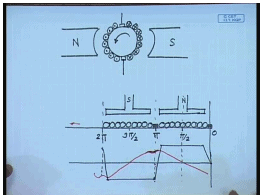So, in a dc machine let us say, dc generator this is the field poles, North Pole and South Pole. This is the brush position, nominal brush position the interpolar region and let us say the machine is rotating in this direction as a generator.

These are the armature conductors, the polarity of the currents in such a direction which will oppose the rotation. If we take a develop diagram of this, which is cut it open, looks somewhat like this, for simplicity we have assumed two pole machine. This is the North Pole, this is the South Pole. So, these are the armature one brush is here, the other brush is here, the flux density wave form due to the armature due to the field is trapezoidal. While the armature reaction flux is such that, it produces a flux density of the whole left behind, which means moving in this direction. So, produces a residual flux density at the brush position, which induces a voltage in the coil undergoing commutation and creates problem with the commutation.

(Refer Slide Time: 07:50)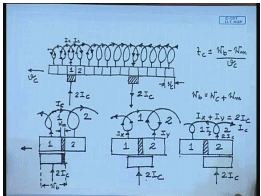We will see what kind of problem may arise. For that just look at the developed diagram of a armature in the commutator segments. If this is these are the commutators each commutator connects to two windings, two coils. So, let us say, at any time a brush is in contact with commutator number 1, carrying the current into it and the other brush let us say it is here one pole each apart carrying out the same current. So, the brush current enters into the coil divides into two parts and flows in this manner. Let us say this individual coil currents are I c. This is I c this is also I c, therefore the total brush current entering is 2 I c.

Similarly, 2 I c will be flowing out here. It is to be same that at the position of the brush the direction of circulation of the current in the coil reverses and between two brushes the coil circulating, the current circulating the same direction. Now, let us say this commutator segment is moving with a velocity of v c in this direction. So, let us look at what happens, when the commutation takes place from commutator number 1 to commutator number 2.

So, let us magnify this position this is commutator number 1 and 2 these are separated by an insulation. Current is entering into the commutator through the brush just this was coil number 1, this is coil number 2. So, at the beginning of the commutation process begins, when the leading edge of this brush coincides with the end of the insulation between the two successive commutators. Let us also define if you other quantities for example, let us say the width of the one commutator segment is W c.

This is W c, width of commutator segment is W c, width of the mica insulation here is W m and width of the brush is W b. In this particular case we will assume W b equal to W c plus W m although it is not necessary, in many cases brush widths are more. After some time the position of the commutator in the brasses will be as follows. This is the mica insulation; the brush should have moved connecting both the commutators. This current is still I c, we see that, this current is still 2 I c, we see that during this position these two commutator segments are shorted by the brush.

These coil one is now shorted by the brush, in the beginning coil current one was I c in this direction. This 2 I c divides into this two by let us say I y and I x. So, we have I x plus I y equal to 2 I c. In the process of commutation this current will change, the current through this coil which was I c previously it will change to minus I c. Let us see how and at the end of commutation, the commutation process will end, when the brush has completely left commutator segment 1, the current flowing still in this direction. This was coil 1, this is coil 2.

So, once it has left, this current will now be 2 I c, this current will be I c and this current will be I c so, we see if we look at coil current 1, coil 1 current see in the beginning of the commutation. It was I c flowing from left to right at the end of commutation still becomes I c, but from right to left. So, in the mean time during the shorting period the current in coil 1 changes from plus I c to minus I c. And the time taken can be easily calculated by the commutation time t c equal to during commutation the brush has moved by an amount W b minus W m by v c. During this period the current in coil one must change from if we assume this direction to be positive from plus I c to minus I c.

(Refer Slide Time: 21:19)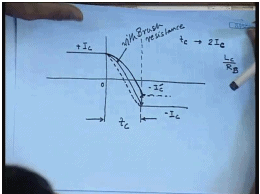Graphically we can show it in this manner, let us say, this is the instant at which commutation begin at this time the current in coil 1 was plus I c. And let us say this is the time when the commutation ends this time being t c commutation time. During this period current changes to at this instance current changes to minus I c, the question remains how the current change here? Ideally we would like the current to change linearly at constant rate from plus I c to minus I c, this is called the ideal commutation characteristics, but in practice it is never so, there are several reasons for these.

In some cases, even when commutator segment 1, in commutator segment the brush segment has left commutator segment 1 as moved to commutator segment 2, the current has not fully reversed, but it has reversed only partially . So, may be it has reached a value of minus I c dash. If that is the case then, when contact with commutator segment 1 breaks at the end of commutation then, there will be a sparking the amount of sparking current will be this. This is called under commutation that is the commutation has no completed before the brush has moved from one commutator segment to the next commutator segment.

Now, this is not desirable since the sparking destroys the commutator surface may also lead to fire hazard. So, this has to be minimized, but let us first find out what are the reasons for under commutation. First is the self inductance of the coil, we see that the current changes from in time t c, current changes from plus I c to minus I c. So, the net change in current is 2 I c. Now the coil undergoing commutation has some leakage inductance, this is mostly due to the overhang part of each coil. So, a coil will have a leakage inductance of L c.

So, this leakage inductance will delay the change in current in the coil undergoing commutation. Not only the leakage inductance of the coil due to armature reaction we have seen that the armature reaction flux. Armature reaction flux leaves a residual flux at the interpolar axis which is of the same polarity as the whole left behind in the generating mode. So, there will be a voltage induced in the coil undergoing commutation which will prevent or delay the change in the current in the coil undergoing commutation leading to under commutation.

Actually we have said, that the effect of under commutation is to produce spark this is to be avoided. There are several ways of mitigating the effect of under commutation one is to use additional resistance in the form of brush contact resistance in the coil undergoing commutation. Let us see if the brush resistance along with the contact resistance is large enough then the time constant formed by the leakage inductance of the coil and the brush contact resistance may be small, which will hasten the change in coil undergoing commutation and we will reduce spark.

This is with brush resistance, this is called resistance commutation, but this cannot completely eliminate the sparking. For that, this is necessary to neutralise the residual flux at the interpolar region. There are different ways of doing it one is the so, called giving a brush lead. If instead of putting the brush actually at the interpolar region if we shift it, lead it that is moving the deduction of rotation in the generating mode. What will happen is that.

In the process of rotation the induced voltage in the coils will change to the polarity of the pole ahead before it reaches the brush. So, there will be a voltage induced in the coil undergoing commutation, which will assist or hustle the change in current in the coil undergoing commutation. So, this can mitigate to some extent the problem of under commutation; however, as we have seen earlier that giving a brush lead creates a demagnetising m m f along the direct axis that is the pole axis.

Because once you have shift the brushes the polarity of the currents will there will change. And if you have given a brush lead of beta then the conductors between the angle two beta will produce a demagnetising flux, which will tend to reduce the main flux ,this is called the demagnetising ampere turns. And it is directly proportional to the brush lead given. So, although giving a shift to the brush, lead to the brush may help commutation it will have a demagnetising effect on the main flux which can be of course, compensated by increasing the ampere turns on the main flux. Another approach of providing additional ampere turns is by providing what we call the interpoles.

(Refer Slide Time: 30:41)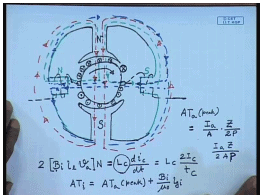The interpoles are short poles provided between two main poles. These are the main poles; let us say this is the armature. These are the brushes, the main flux adopt this flowing this direction. This is the main flux; let us say the generating mode the direction of the rotation is this, in which case if we take two armature conductors the polarity of the currents will be this dot, this cross. In which case in the generating mode the m m f produced by the armature coils because all cross here and all dots here.

So, the armature m m f produced the armature flux, armature m m f, armature reaction flux will tend to flow in this direction. In other words the armature m m f will produce a virtual north pole at the interpolar region. A flux that is equivalent to a north pole that is the pole left behind in the generating mode. See if we want to cancel this flux then in the interpolar region we can put an actual south pole that is, this South Pole and a north pole here.

So that, the flux produced by this north pole will cancel the armature reaction, not only it will cancel the armature reaction flux. But it will also produce a flux which will generate the voltage in the coil undersigning commutation of the polarity in that, it will have under the pole ahead in the generating mode, which will assist commutation. So, these are called interpoles, interpoles are short and narrow pole structures placed at the interpolar location in order to compensate the interpolar armature reaction flux. And since the interpole is suppose to compensate the armature reaction flux; these are excited by the armature current. That is, it should the interpoles are excited by the armature current that is the coil on the interpole is connected in series with the armature winding. So, that at on load conditions it can cancel the armature reaction flux.

Now, let us see, how much this flux density at the interpolar region is required, the voltage induced in the coil at the interpolar location will be two times the interpolar flux, desired interpolar flux density B I into the length of the conductor into velocity of the armature into number of turns. This should cancel the voltage due to the self inductance of the coil undergoing commutation.

So, ideally what we should have is that? The interpoles will not only compensate the armature reaction ampere turns, but in addition it should also generate a flux density at the interpolar location. Such that the voltage induced due to the coil undergoing commutation due to that flux density cancels, the voltage induced by the leakage inductance of the coil undergoing commutation.

However there is a practical difficulty, because finding out the value of the leakage inductance is not easy and this flux density as to be set by some what a trial and error method. Once we have find out the required flux density B I then the required ampere turns for the interpole can be calculated as follows ampere turns. The interpole should be equal to peak armature reaction ampere turns plus the ampere turns required to generate a flux density of B I at the inter pole.

Since we neglect the ampere turns required to circulate flux in the core this is given by. where B I is the flux density, required flux density at the interpole of the desired polarity (( )) is the permeability, l g is the length of air gap at the interpole, total length of the air gap at the interpole and of course, mu naught is the permeability of the free space.

Now, peak ampere turn we know per pole is given by equal to. If the armature current is I a, then the current in each parallel path is I a by A, if there are z number of conductors then turns per pole is z by 2 p. So, the peak ampere turns is given by I a z by 2 A P. So, form which we will be able to calculate the required ampere turns of the interpoles and the corresponding number of turns in the interpoles. So, let us try to solve some problems, involving the commutation and induce voltage interpoles etcetera of a d c machine.

(Refer Slide Time: 45:30)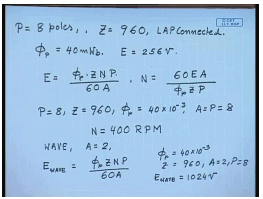Let us say that, we have a d c machine with 8 poles, there are 960 that is, P equal to 8 there are 960 conductors that is z equal to 960, which are lap connected. The flux per pole of the machine is 40 milliwabor, if we want a no load induce voltage E of 256 volts, what should be the speed of rotation? This is the straight forward application of the formula of the induced voltage which is given by E equal to phi p into z N P by 60 A, where phi p is the flux per pole, z is total number of conductor, N is the speed of the rotation in R P M, P is the number of poles and A is the number of parallel paths.

In other words N can be written as 60 E A divided by phi p z P, in the given problem P equal to 8, z equal to 960, phi p equal to 40 into10 to the power minus 3. And since the machine, armature is lap connected, A is equal to P equal to 8 therefore, the answer will be N equal to substituting all these values we get, N equal to 400 R P M. It will be interesting to find out, if I run the machine at the same 400 R P M, but change the armature conductor connection to wave, what will be the induced voltage.

We know for wave connection A equal to always 2. So, for wave, E wave will be equal to phi p z N P by 60 A. Substituting the values phi p equal to 40 into 10 to the power minus 3, z equal to 960, A equal to 2, P equal to 8, we will get E wave equal to 1024volts. So, the induced voltage in a wave connected machine at the same speed will be much higher.

(Refer Slide Time: 50:17)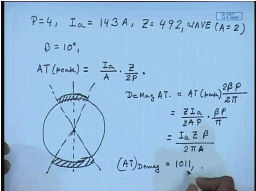Now, let us look at the problem of the commutation.

Let us say that, we have a machine with 4 pole, which carries a full load armature current I a of 143 amperes, there are total 492 wave connected conductors. In order to mitigate the condition problem a phase set beta of N degrees is applied to the brushes, what will be the demagnetising ampere turns? We have seen in our previous class that, armature reaction flux A T peak is given by I a by A, which is the coil current mu 2, z by 2 p.

If we look at the picture, let us say this is the nominal magnetic neutralisation. If I give a phase lead, the brushes of beta, we have seen earlier that all the conductors inside the angle 2 beta produce demagnetising ampere turns. So, the demagnetising ampere turns De Mag A T is equal to A T peak beta. And is the 2 beta is the angle over which, the conductors will demagnetise and between two poles the separation is 2 pi by p.

So, demagnetising A T will be z I a by A into 2 P into beta P by pi or this will be equal to z I a beta by 2 pi A. Substituting the given values, A is the number of parallel paths and in this case A equal to 2, substituting the values we get demagnetising, A T demagnetising equal to 1011. If the field current is known then, we can find out the number of extra terms that will be required on the field winding in order to cancel the demagnetising A T. So, this divided by field current will give you the number of extra field turn required for d c machine.

Thank you.

Offer running on EduRev: Apply code STAYHOME200 to get INR 200 off on our premium plan EduRev Infinity!

61 docs|20 tests

,

,

,

,

,

,

,

,

,

,

,

,

,

,

,

,

,

,

,

,

,

;